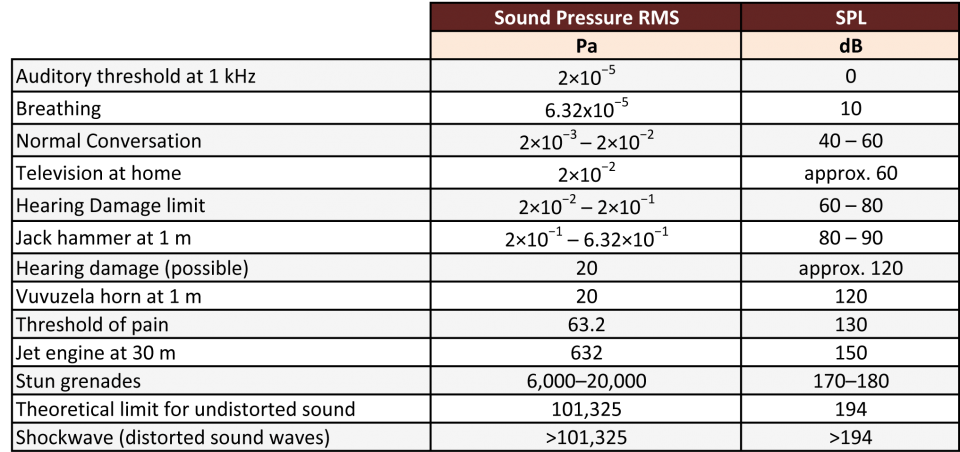Categories

# Grandma Moon Speaks: RMS and the Decibel

Topics Covered:

• Root Mean Square
• The Decibel
• Common dB Values

Day has its eyes; night has its ears – Japanese ProverbIn the above drawing, the numbers represent:

1. Silence
2. Audible sound
3. Atmospheric pressure
4. Sound pressure

105 Pa is the pressure at sea level, or 1 atmosphere approximately. We have already seen 20 µPa above this is the threshold for hearing.

What you notice from the above graph is that sound can be both ‘positive’ and ‘negative’, in the wave sense. To average a particular value, one of the traditional methods is using what is called the Root Mean Square method, or RMS.If f(t) is a function that represents values at different intervals of time, between T1 and T2, then the above formula gives the method to find the root mean square of all of them, also sometimes called the quadratic mean.

For a simple sine wave, the RMS value is A/?2, where A is the amplitude of the wave.

Enough of all these formulas. The threshold of hearing is correctly 20 µPa RMS. This value is taken as a ‘zero’ reference to measure the pressure of sound, called the Sound Pressure Level (SPL).Where
Lp is the SPL
Prms is the root-mean-square sound pressure
Pref is the reference sound pressure, commonly 20 µPa

Dr. Optoglass might have mentioned that using a logarithmic scale allows one to cram in more values in a given space over a linear scale. The human range of hearing is pretty large indeed, and its response to stimulus matches a logarithmic scale more than it does a linear scale.

For this reason, it is customary to use a logarithmic scale, and this measurement unit is called a decibel (dB). Deci is 10 (as in decimal) since this is log to the base 10. Bel is in honor of Alexander Graham Bell, the dude who started this all. This is why d is in lower case while B is in upper case.

0 dB corresponds to 20 µPa. This is like matching the 0 level of an inch and centimeter scale. Anything below 0 dB is not of much value when talking about the human audible range. What about the upper limit?

How high can the dB value go before our ears burst? Here’s a list:As you can see, the range in Pa is extremely large, while in dB it is easier to read. However, it is easy to forget that a small change in dB usually means a big difference to our ears!

Takeaways:

• Root Mean Square or RMS is a way to average values that are part of complex waves.
• The unit of Sound Pressure Level (SPL) is Decibel, or dB.
• The threshold of hearing (20 µPa) is generally equated to an SPL of 0 dB.